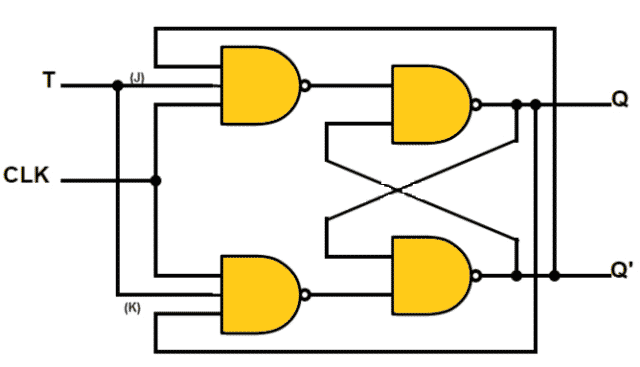Thursday, November 30, 2023
HomeDigital TechnologyT FLIP FLOP - Construction/ Design, Working Principle and Applications

# T FLIP FLOP – Construction/ Design, Working Principle and Applications

T Flip Flop is one among the many basic types of Flip-Flop’s available. The basic functionality of Flip-Flop is to store information. Flip-Flops and latches are the basic building blocks of Digital Electronics communication. It finds its use in counter design and in sequential circuits design where switching  operation is necessary. This post will give you a brief idea about what is T Flip Flop, it’s construction/ design, working principle, applications, advantages and disadvantages.

## What is T Flip Flop

This Flip-Flop is similar to JK Flip Flop, where the inputs of J and K are joined together and made as single input. It has single input along with clock input. It is also referred as Toggle Flip-Flop, toggle means to change i.e. switching to opposite state. The resulting output is half the frequency of the signal to the T input.Fig. 1 – Circuit Symbol of T Flip Flop

## Construction/ Design of T Flip Flop

It can be designed using 3 methods. They are: –

• Using SR (Set-Reset) Flip-Flop
• Using D (Data) Flip-Flop
• Using JK Flip-Flop

### T Flip-Flop Using SR Flip-Flop

• It is constructed using AND gates as input to NOR gate SR latch.
• Input of AND gates are fed back with output Q and Q΄ to each AND gate.
• Toggle input (T) is connected to both the AND gates as input.
• Clock signal (CLK) is also connected in common with AND gates.
• A pulse of narrow triggers is provided as input at T, which changes the output state of Flip-Flop.

Below is the circuit diagram of Toggle Flip-Flop using SR latch.Fig. 2 – T Flip-Flop using SR Latch

### T  Flip-Flop using D Flip-Flop

• In this type of design, the output of QPREV (Previous state of Q) is XORed with input (T) and given at input D.
• At every positive edge when T=0, D=Q and this state will remain same.
• When T=1 at positive edge clock, D=Q’ and will remain unchanged.
• It retains its present state at T=0 and toggles it when T=1.

Below is the circuit diagram for T FlipFlop using D Flip-Flop.Fig. 3 – T Flip-Flop using D Flip flop

### T Flip Flop using JK Flip-Flop

• Here J and K input of the JK Flip-Flop is connected together and given to the T input.
• If the T input is in 0 state (i.e., J = K = 0) prior to a clock pulse, the Q output will not change with the clock pulse.
• If the T input is in 1 state (i.e., J = K = 1) prior to a clock pulse, the Q output will change to Q’ with the clock pulse.
• We can conclude that if T = 1 and the device is clocked, then the output toggles its state.

Below is the circuit diagram of T  FlipFlop using JK Flip-Flop.Fig. 4 – T Flip-Flop using JK Flip-Flop

## Working Principle

This (T) Flip-Flop is a synchronous device, where high to low or low to high transitions is passed through clock signal which changes the output state of Flip-Flop.

Let us take an example of T FlipFlop made of NAND SR Latch.Fig. 5 – T Flip-Flop using NAND SR LatchFig. 6 – T Flip-Flop Truth Table

• If output Q is 0, the above NAND is enabled and the below one is disabled, the S input mode will be in SET state i.e. Q =1
• If output Q is 1, the above NAND is disabled and the below one is enabled, the R input mode will be in RESET state i.e. Q =0
• When T input is low,
1. T = 0, present state = 0, next state = 0
2. T = 1, present state = 1, next state = 1
• When T input is high, during positive transition of clock signal
1. T = 1, present state = 0, next state = 1
2. T = 1, present state = 1, next state = 0
• The trigger changes the set and reset inputs alternatively, so the flip flop toggles. The T flipflop is called as “Frequency divider circuit” because it produces the output at half of the input frequency.

## Applications of T Flip-Flop

The applications are:

• Used as bounce elimination switch
• Used as frequency divider circuit that means divides the frequency of periodic wave forms
• Used as data storage where the Flip-Flops are connected in series in which each Flip-Flop stores one-bit information
• Used as digital counters, it counts pulses or events and it can be made by connecting series of Flip-Flops

• These Flip-Flops has a toggle input and a clock. When a clock is triggered it inverts the value of Flip-Flops.
• They are good for counters.
• In Minecraft piston, they are used as they are very small in size.

• The state of Flip-Flop is known only when the previous state is known.
• They are not available as IC’s, hence they are constructed using JK Flip-Flop, D Flip-Flop etc.
```Also Read:
Filters – Classification, Characteristics, Types, Applications & Advantages
Waveguide – Classification, Modes, How it Works, Application, Advantage
```Laxmi Ashrit
Laxmi is a B.E (Electronics & Communication) and has work experience in RelQ Software as Test Engineer and HP as Technical support executive. She is an author, editor and partner at Electricalfundablog.
RELATED ARTICLES